Get inspired by the success stories of our students in IIT JAM MS, ISI  MStat, CMI MSc DS.  Learn More

# ISI MStat PSB 2010 Problem 10 | Uniform ModifiedThis is a very elegant sample problem from ISI MStat PSB 2010 Problem 10. It's mostly based on propertes of uniform, and its behaviour when modified . Try it!

## Problem- ISI MStat PSB 2010 Problem 10

Let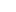be a random variable uniformly distributed over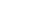,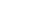, and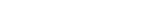.

(a) Find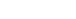.

(b) Let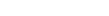be a random sample from the above distribution with unknown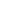. Find two distinct unbiased estimators of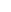, as defined in (a), based on the entire sample.

### Prerequisites

Uniform Distribution

Law of Total Expectation

Unbiased Estimators

## Solution :

Well, this is a very straight forward problem, where we just need to be aware the way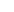is defined.

As, we need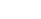and by definition of, we clearly see thatis dependent inwhere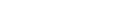.

So, using Law of Total Expectation,, why ??

Also, conditional pdf of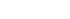is,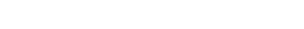. [where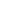is the pdf of].

the other conditional pdf is also same due to symmetry.(Verify!!).

So,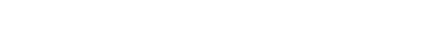.

hence,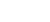.

Now, for the next part, one trivial unbiased estimator ofis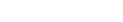(based on the given sample). So,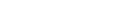is an obvious unbiased estimator of.

For another we need to change our way of looking on conventional way and look for the order statistics, since we know that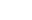is sufficient for.(Don't Know ?? Look for Factorization Theorem .)

So, verify that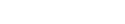.

Hence,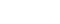is another unbiased estimator of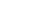. So,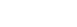is also another unbiased estimator ofa defined in (a).

Hence the solution concludes.

## Food For Thought

Let us think about some unpopular but very beautiful relationship between discrete random variables besides the Universality of uniform. Letbe a discrete random variable with cdf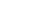and define the random variable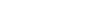.

Can you verify that,is stochastically greater that a uniform(0,1) random variable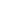. i.e.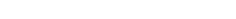for all,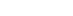,, for some,.

Hint: Draw a typical picture of a discrete cdf, and observe the jump points ! you may jump to the solution!! Think it over.

## Subscribe to Cheenta at Youtube

This is a very elegant sample problem from ISI MStat PSB 2010 Problem 10. It's mostly based on propertes of uniform, and its behaviour when modified . Try it!

## Problem- ISI MStat PSB 2010 Problem 10

Letbe a random variable uniformly distributed over,, and.

(a) Find.

(b) Letbe a random sample from the above distribution with unknown. Find two distinct unbiased estimators of, as defined in (a), based on the entire sample.

### Prerequisites

Uniform Distribution

Law of Total Expectation

Unbiased Estimators

## Solution :

Well, this is a very straight forward problem, where we just need to be aware the wayis defined.

As, we needand by definition of, we clearly see thatis dependent inwhere.

So, using Law of Total Expectation,, why ??

Also, conditional pdf ofis,. [whereis the pdf of].

the other conditional pdf is also same due to symmetry.(Verify!!).

So,.

hence,.

Now, for the next part, one trivial unbiased estimator ofis(based on the given sample). So,is an obvious unbiased estimator of.

For another we need to change our way of looking on conventional way and look for the order statistics, since we know thatis sufficient for.(Don't Know ?? Look for Factorization Theorem .)

So, verify that.

Hence,is another unbiased estimator of. So,is also another unbiased estimator ofa defined in (a).

Hence the solution concludes.

## Food For Thought

Let us think about some unpopular but very beautiful relationship between discrete random variables besides the Universality of uniform. Letbe a discrete random variable with cdfand define the random variable.

Can you verify that,is stochastically greater that a uniform(0,1) random variable. i.e.for all,,, for some,.

Hint: Draw a typical picture of a discrete cdf, and observe the jump points ! you may jump to the solution!! Think it over.

## Subscribe to Cheenta at Youtube

This site uses Akismet to reduce spam. Learn how your comment data is processed.

### Knowledge Partner